## Exercise 4.1 page no: 4.3

1. Write down the numerator of each of the following rational numbers:
(i) (-7/5)
(ii) (14/-4)
(iii) (-17/-21)
(iv) (8/9)
(v) 5
Solution:
(i) Given (-7/5)
Numerator of (-7/5) is -7
(ii) Given (14/-4)
Numerator of (14/-4) is 1
(iii) Given (-17/-21)
Numerator of (-17/-21) is -17
(iv) Given (8/9)
Numerator of (8/9) is 8
(v) Given 5
Numerator of 5 is 5
2. Write down the denominator of each of the following rational numbers:
(i) (-4/5)
(ii) (11/-34)
(iii) (-15/-82)
(iv) 15
(v) 0
Solution:
(i) Given (-4/5)
Denominator of (-4/5) is 5
(ii) Given (11/-34)
Denominator of (11/-34) is -43
(iii) Given (-15/-82)
Denominator of (15/-82) is -82
(iv) Given 15
Denominator of 15 is 1
(v) Given 0
Denominator of 0 is any non-zero integer
3. Write down the rational number whose numerator is (-3) × 4, and whose denominator is (34 – 23) × (7 – 4).

Solution:
Given numerator = (-3) × 4 = -12
Denominator = (34 – 23) × (7 – 4)
= 11 × 3 = 33
Therefore the rational number = (-12/33)
4. Write down the rational numbers as integers: (7/1), (-12/1), (34/1), (-73/1), (95/1)
Solution:
Given (7/1), (-12/1), (34/1), (-73/1), (95/1)
Integers of (7/1), (-12/1), (34/1), (-73/1), (95/1) are 7, -12, 34, -73, 95
5. Write the following integers as rational numbers: -15, 17, 85, -100
Solution:
Given -15, 17, 85, -100
The rational numbers of given integers are (-15/1), (17/1), (85/1) and (-100/1)
6. Write down the rational number whose numerator is the smallest three digit number and denominator is the largest four digit number.
Solution:
Smallest three digit number = 100
Largest four digit number = 9999
Therefore the rational number is = 100/9999
7. Separate positive and negative rational numbers from the following rational numbers:
(-5/-7), (12/-5), (7/4), (13/-9), 0, (-18/-7), (-95/116), (-1/-9)
Solution:
Given (-5/-7), (12/-5), (7/4), (13/-9), 0, (-18/-7), (-95/116), (-1/-9)
A rational number is said to be positive if its numerator and denominator are either positive integers or both negative integers.
Therefore positive rational numbers are: (-5/-7), (-18/-7), (7/4), (-1/-9)
A rational number is said to be negative integers if its numerator and denominator are such that one of them is positive integer and another one is a negative integer.
Therefore negative rational numbers are: (12/-5), (13/-9), (-95/116)
8. Which of the following rational numbers are positive:
(i) (-8/7)
(ii) (9/8)
(iii) (-19/-13)
(iv) (-21/13)
Solution:
Given (-8/7), (9/8), (-19/-13), (-21/13)
A rational number is said to be positive if its numerator and denominator are either positive integers or both negative integers.
Therefore the positive rational numbers are (9/8) and (-19/-13)
9. Which of the following rational numbers are negative:
(i) (-3/7)
(ii) (-5/-8)
(iii) (9/-83)
(iv) (-115/-197)
Solution:
Given (-3/7), (-5/-8), (9/-83), (-115/-197)
A rational number is said to be negative integers if its numerator and denominator are such that one of them is positive integer and another one is a negative integer.
Therefore negative rational numbers are (-3/7) and (9/-83)

## Exercise 4.2 page no: 4.8

1. Express each of the following as a rational number with positive denominator.
(i) (-15/-28)
(ii) (6/-9)
(iii) (-28/-11)
(iv) (19/-7)
Solution:
(i) Given (-15/-28)
Multiplying both numerator and denominator we can rational number with positive denominator.
(-15/-28) = (-15/-28) × -1
= (15/28)
(ii) Given (6/-9)
Multiplying both numerator and denominator we can rational number with positive denominator.
(6/-9) = (6/-9) × -1
= (-6/9)
(iii) Given (-28/-11)
Multiplying both numerator and denominator we can rational number with positive denominator.
(-28/-11) = (-28/-11) × -1
= (28/11)
(iv) Given (19/-7)
Multiplying both numerator and denominator we can rational number with positive denominator.
(19/-7) = (19/-7) × -1
= (-19/7)
2. Express (3/5) as a rational number with numerator:
(i) 6
(ii) -15
(iii) 21
(iv) -27
Solution:
(i) Given (3/5)
To get numerator 6 we have to multiply both numerator and denominator by 2
Then we get, (3/5) × (2/2) = (6/10)
Therefore (3/5) as a rational number with numerator 6 is (6/10)
(ii) Given (3/5)
To get numerator -15 we have to multiply both numerator and denominator by -5
Then we get, (3/5) × (-5/-5)
= (-15/-25)
Therefore (3/5) as a rational number with numerator -15 is (-15/-25)
(iii) Given (3/5)
To get numerator 21 we have to multiply both numerator and denominator by 7
Then we get, (3/5) × (7/7)
= (21/35)
Therefore (3/5) as a rational number with numerator 21 is (21/35)
(iv) Given (3/5)
To get numerator -27 we have to multiply both numerator and denominator by -9
Then we get, (3/5) × (-9/-9)
= (-27/-45)
Therefore (3/5) as a rational number with numerator -27 is (-27/-45)
3. Express (5/7) as a rational number with denominator:
(i) -14
(ii) 70
(iii) -28
(iv) -84
Solution:
(i) Given (5/7)
To get denominator -14 we have to multiply both numerator and denominator by -2
Then we get, (5/7) × (-2/-2)
= (-10/-14)
Therefore (5/7) as a rational number with denominator -14 is (-10/-14)
(ii) Given (5/7)
To get denominator 70 we have to multiply both numerator and denominator by -2
Then we get, (5/7) × (10/10)
= (50/70)
Therefore (5/7) as a rational number with denominator 70 is (50/70)
(iii) Given (5/7)
To get denominator -28 we have to multiply both numerator and denominator by -4
Then we get, (5/7) × (-4/-4)
= (-20/-28)
Therefore (5/7) as a rational number with denominator -28 is (-20/-28)
(iv) Given (5/7)
To get denominator -84 we have to multiply both numerator and denominator by -12
Then we get, (5/7) × (-12/-12)
= (-60/-84)
Therefore (5/7) as a rational number with denominator -84 is (-60/-84)
4. Express (3/4) as a rational number with denominator:
(i) 20
(ii) 36
(iii) 44
(iv) -80
Solution:
(i) Given (3/4)
To get denominator 20 we have to multiply both numerator and denominator by 5
Then we get, (3/4) × (5/5)
= (15/20)
Therefore (3/4) as a rational number with denominator 20 is (15/20)
(ii) Given (3/4)
To get denominator 36 we have to multiply both numerator and denominator by 9
Then we get, (3/4) × (9/9)
= (27/36)
Therefore (3/4) as a rational number with denominator 36 is (27/36)
(iii) Given (3/4)
To get denominator 44 we have to multiply both numerator and denominator by 11
Then we get, (3/4) × (11/11)
= (33/44)
Therefore (3/4) as a rational number with denominator 44 is (33/44)
(iv) Given (3/4)
To get denominator -80 we have to multiply both numerator and denominator by -20
Then we get, (3/4) × (-20/-20)
= (-60/-80)
Therefore (3/4) as a rational number with denominator -80 is (-60/-80)
5. Express (2/5) as a rational number with numerator:
(i) -56
(ii) 154
(iii) -750
(iv) 500
Solution:
(i) Given (2/5)
To get numerator -56 we have to multiply both numerator and denominator by -28
Then we get, (2/5) × (-28/-28)
= (-56/-140)
Therefore (2/5) as a rational number with numerator -56 is (-56/-150)
(ii) Given (2/5)
To get numerator 154 we have to multiply both numerator and denominator by 77
Then we get, (2/5) × (77/77)
= (154/385)
Therefore (2/5) as a rational number with numerator 154 is (154/385)
(iii) Given (2/5)
To get numerator -750 we have to multiply both numerator and denominator by -375
Then we get, (2/5) × (-375/-375)
= (-750/-1875)
Therefore (2/5) as a rational number with numerator -750 is (-750/-1875)
(iv) Given (2/5)
To get numerator 500 we have to multiply both numerator and denominator by 250
Then we get, (2/5) × (250/250)
= (500/1250)
Therefore (2/5) as a rational number with numerator 500 is (500/1250)
6. Express (-192/108) as a rational number with numerator:
(i) 64
(ii) -16
(iii) 32
(iv) -48
Solution:
(i) Given (-192/108)
To get numerator 64 we have to divide both numerator and denominator by -3
Then we get, (-192/108) ÷ (-3/-3)
= (64/-36)
Therefore (-192/108) as a rational number with numerator 64 is (64/-36)
(ii) Given (-192/108)
To get numerator -16 we have to divide both numerator and denominator by 12
Then we get, (-192/108) ÷ (12/12)
= (-16/9)
Therefore (-192/108) as a rational number with numerator -16 is (-16/9)
(iii) ) Given (-192/108)
To get numerator 32 we have to divide both numerator and denominator by -6
Then we get, (-192/108) ÷ (-6/-6)
= (32/-18)
Therefore (-192/108) as a rational number with numerator 32 is (32/-18)
(iv) Given (-192/108)
To get numerator -48 we have to divide both numerator and denominator by 4
Then we get, (-192/108) ÷ (4/4)
= (-48/27)
Therefore (-192/108) as a rational number with numerator -48 is (-48/27)
7. Express (169/-294) as a rational number with denominator:
(i) 14
(ii) -7
(iii) -49
(iv) 1470
Solution:
(i) Given (169/-294)
To get denominator 14 we have to divide both numerator and denominator by -21
Then we get, (169/-294) ÷ (-21/-21)
= (-8/14)
Therefore (169/-294) as a rational number with denominator 14 is (-8/14)
(ii) Given (169/-294)
To get denominator -7 we have to divide both numerator and denominator by 42
Then we get, (169/-294) ÷ (42/42)
= (4/-7)
Therefore (169/-294) as a rational number with denominator -7 is (4/-7)
(iii) Given (169/-294)
To get denominator -49 we have to divide both numerator and denominator by 6
Then we get, (169/-294) ÷ (6/6)
= (28/-49)
Therefore (169/-294) as a rational number with denominator -49 is (28/-49)
(iv) Given (169/-294)
To get denominator 1470 we have to multiply both numerator and denominator by -5
Then we get, (169/-294) × (-5/-5)
= (-840/1470)
Therefore (169/-294) as a rational number with denominator 1470 is (-840/1470)
8. Write (-14/42) in a form so that the numerator is equal to:
(i) -2
(ii) 7
(iii) 42
(iv) -70
Solution:
(i) Given (-14/42)
To get numerator -2 we have to divide both numerator and denominator by 7
Then we get, (-14/42) ÷ (7/7)
= (-2/6)
Therefore (-14/42) as a rational number with numerator -2 is (-2/6)
(ii) Given (-14/42)
To get numerator 7 we have to divide both numerator and denominator by -2
Then we get, (-14/42) ÷ (-2/-2)
= (7/-21)
Therefore (-14/42) as a rational number with numerator -14 is (-14/21)
(iii) Given (-14/42)
To get numerator 42 we have to multiply both numerator and denominator by -3
Then we get, (-14/42) × (-3/-3)
= (42/-126)
Therefore (-14/42) as a rational number with numerator 42 is (42/-126)
(iv) Given (-14/42)
To get numerator -70 we have to multiply both numerator and denominator by 5
Then we get, (-14/42) × (5/5)
= (-70/210)
Therefore (-14/42) as a rational number with numerator -70 is (-70/210)
9. Select those rational numbers which can be written as a rational number with numerator 6:
(1/22), (2/3), (3/4), (4/-5), (5/6), (-6/7), (-7/8)
Solution:
Given rational numbers that can be written as a rational number with numerator 6 are:
Consider (1/22)
On multiplying by 6, (1/22) can be written as
(1/22) = (6/132)
Consider (2/3)
On multiplying by 3, (2/3) can be written as
(2/3) = (6/9)
Consider (3/4)
On multiplying by 2, (3/4) can be written as
(3/4) = (6/8)
Consider (-6/7)
On multiplying by -1, (-6/7) can be written as
(-6/7) = (6/-7)
Therefore rational numbers that can be written as a rational number with numerator 6 are (1/22), (2/3), (3/4) and (-6/7)
10. Select those rational numbers which can be written as rational number with denominator 4:
(7/8), (64/16), (36/-12), (-16/17), (5/-4), (140/28)
Solution:
Given rational numbers that can be written as a rational number with denominator 4 are:
(7/8) = (3.5/4) (On dividing both denominator and denominator by 2)
(64/16) = (16/4) (On dividing both denominator and numerator by 4)
(36/-12) = (-12/4) (On dividing both denominator and numerator by -3)
(5/- 4) = (- 5/4) (On multiplying both denominator and numerator by -1)
(140/28) = (20/4) (On dividing both numerator and denominator by 7)
11. In each of the following, find an equivalent form of the rational number having a common denominator:
(i) (3/4) and (5/12)
(ii) (2/3), (7/6) and (11/12)
(iii) (5/7), (3/8), (9/14) and (20/21)
Solution:
(i) Given (3/4) and (5/12)
On multiplying both numerator and denominator by 3
(3/4) = (3 × 3)/ (4 × 3) = (9/12)
Equivalent forms with same denominators are (9/12) and (5/12)
(ii) Given (2/3), (7/6) and (11/12)
On multiplying both numerator and denominator by 4
(2/3) = (2 × 4)/ (3 × 4) = (8/12)
And (7/6) = (7 × 2)/ (6 × 2) = (14/12)
Equivalent forms are (8/12), (14/12) and (11/12) having same denominators
(iii) Given (5/7), (3/8), (9/14) and (20/21)
(5/7) = (5 × 24)/ (7 × 24) = (120/168) [on multiplying both numerator and denominator by 24]
(3/8) = (3 × 21)/ (8 × 21) = (63/168) [on multiplying both numerator and denominator by 21]
(9/14) = (9 × 12)/ (14 × 12) = (108/168) [on multiplying both numerator and denominator by 12]
(20/21) = (20 × 8)/ (21×8) = (160/168) [on multiplying both numerator and denominator by 8]
Forms are (120/168), (63/168), (108/168) and (160/168) having same denominators.

## Exercise 4.3 page no: 4.12

1. Determine whether the following rational numbers are in the lowest form or not:
(i) (65/84)
(ii) (-15/32)
(iii) (24/128)
(iv) (-56/-32)
Solution:
(i) Given (65/84)
Here we can observe that 65 and 84 have no common factor their HCF is 1.
Thus, (65/84) is in its lowest form.
(ii) Given (-15/32)
Here we can observe that -15 and 32 have no common factor i.e., their HCF is 1.
Thus, (-15/32) is in its lowest form.
(iii) Given (24/128)
Here we can observe that HCF of 24 and 128 is not 1.
Thus, given rational number is not in its simplest form.
(iv) Given (-56/-32)
Here we can observe that HCF of 56 and 32 is 8 and also not equal to 1.
Therefore the given rational number is not in its simplest form.
2. Express each of the following rational numbers to the lowest form:
(i) (4/22)
(ii) (-36/180)
(iii) (132/-428)
(iv) (-32/-56)
Solution:
(i) Given (4/22)
We know that HCF of 4 and 22 is 2
By dividing the given number by its HCF we get
(4 ÷ 2/22 ÷ 2) = (2/11)
Therefore (2/11) is the simplest form of the given number
(ii) Given (-36/180)
We know that HCF of 36 and 180 is 36
By dividing the given number by its HCF we get
(-36 ÷ 36/180 ÷ 36) = (-1/5)
Therefore (-1/5) is the simplest form of the given number
(iii) Given (132/-428)
We know that HCF of 132 and 428 is 4
By dividing the given number by its HCF we get
(132 ÷ 4/-428 ÷ 4) = (33/-107)
Therefore (33/-107) is the simplest form of the given number
(iv) Given (-32/-56)
We know that HCF of 32 and 56 is 8
By dividing the given number by its HCF we get
(-32 ÷ 8/-56 ÷ 8) = (4/7)
Therefore (4/7) is the simplest form of the given number
3. Fill in the blanks:
(i) (-5/7) = (…/35) = (…/49)
(ii) (-4/-9) = (…/18) = (12/…)
(iii) (6/-13) = (-12/…) = (24/…)
(iv) (-6/…) = (3/11) = (…/-55)
Solution:
(i) (-5/7) = (-25/35) = (-35/49)
Explanation:
Given (-5/7) = (…/35) = (…/49)
Here (-5/7) × (5/5) = (-25/35)
And also (-5/7) × (7/7) = (-35/49)
(ii) (-4/-9) = (8/18) = (12/27)
Explanation:
Given (-4/-9) = (…/18) = (12/…)
On multiplying by -2 we get
(-4/-9) × (-2/-2) = (8/18)
Also on multiplying by -3
(-4/-9) × (-3/-3) = (12/27)
(iii) (6/-13) = (-12/26) = (24/-52)
Explanation:
Given (6/-13) = (-12/…) = (24/…)
On multiplying by -2
(6/-13) × (-2/-2) = (-12/26)
Also multiplying by 4
And also (6/-13) × (4/4) = (24/-52)
(iv) (-6/-22) = (3/11) = (-15/-55)
Explanation:
Given (-6/…) = (3/11) = (…/-55)
0n multiplying by -2
(3/11) × (-2/-2) = (-6/-22)
And also on multiplying by -5
(3/11) × (-5/-5) = (-15/-55)

## Exercise 4.4 page no: 4.15

1. Write each of the following rational numbers in the standard form:
(i) (2/10)
(ii) (-8/36)
(iii) (4/-16)
(iv) (-15/-35)
(v) (299/-161)
(vi) (-63/-210)
(vii) (68/-119)
(viii) (-195/275)
Solution:
(i) Given (2/10)
We know that HCF of 2 and 10 is 2
Now dividing the numerator and denominator by HCF i.e. 2, we get:
(2/10) ÷ (2/2) = (1/5)
Therefore (1/5) is the standard form of given number
(ii) Given (-8/36)
We know that HCF of 8 and 36 is 4
Now dividing the numerator and denominator by HCF i.e. 4, we get:
(-8/36) ÷ (4/4) = (-2/9)
Therefore (-2/9) is the standard form of given number
(iii) Given (4/-16)
Here denominator is negative so we have multiply both numerator and denominator by -1
(4/-16) × (-1/-1) = (-4/16)
We know that HCF of 4 and 16 is 4
Now dividing the numerator and denominator by HCF i.e. 4, we get:
(-4/16) ÷ (4/4) = (-1/4)
Therefore (-1/4) is the standard form of given number
(iv) Given (-15/-35)
Here denominator is negative so we have multiply both numerator and denominator by -1
(-15/-35) × (-1/-1) = (15/35)
We know that HCF of 15 and 35 is 4
Now dividing the numerator and denominator by HCF i.e. 5, we get:
(15/35) ÷ (5/5) = (3/7)
Therefore (3/7) is the standard form of given number
(v) Given (299/-161)
Here denominator is negative so we have multiply both numerator and denominator by -1
(299/-161) × (-1/-1) = (-299/161)
The HCF of 299 and 161 is 23
Now dividing the numerator and denominator by HCF i.e. 23, we get:
(-299/161) ÷ (23/23) = (-13/7)
Therefore (-13/7) is the standard form of given number
(vi) Given (-63/-210)
The HCF of 63 and 210 is 21
Now dividing the numerator and denominator by HCF i.e. 21, we get:
(-63/-210) ÷ (21/21) = (-3/-10) = (3/10)
Therefore (3/10) is the standard form of given number
(vi) Given (68/-119)
Here denominator is negative so we have multiply both numerator and denominator by -1
(68/-119) × (-1/-1) = (-68/119)
The HCF of 68 and 119 is 17
Now dividing the numerator and denominator by HCF i.e. 17, we get:
(-68/119) ÷ (17/17) = (-4/7)
Therefore (-4/7) is the standard form of given number
(viii) Given (-195/275)
The HCF of 195 and 257 is 5
Now dividing the numerator and denominator by HCF i.e. 5, we get:
(-165/275) ÷ (5/5) = (-39/55)
Therefore (-39/55) is the standard form of given number

## Exercise 4.5 page no: 4.20

1. Which of the following rational numbers are equal?
(i) (-9/12) and (8/-12)
(ii) (-16/20) and (20/-25)
(iii) (-7/21) and (3/-9)
(iv) (-8/-14) and (13/21)
Solution:
(i) Given (-9/12) and (8/-12)
The standard form of (-9/12) is (-3/4) [on diving the numerator and denominator of given number by their HCF i.e. by 3]
The standard form of (8/-12) = (-2/3) [on diving the numerator and denominator of given number by their HCF i.e. by 4]
Since, the standard forms of two rational numbers are not same. Hence, they are not equal.
(ii) Given (-16/20) and (20/-25)
Multiplying numerator and denominator of (-16/20) by the denominator of (20/-25)
i.e. -25.
(-16/20) × (-25/-25) = (400/-500)
Now multiply the numerator and denominator of (20/-25) by the denominator of
(-16/20) i.e. 20
(20/-25) × (20/20) = (400/-500)
Clearly, the numerators of the above obtained rational numbers are equal.
Hence, the given rational numbers are equal
(iii) Given (-7/21) and (3/-9)
Multiplying numerator and denominator of (-7/21) by the denominator of (3/-9)
i.e. -9.
(-7/21) × (-9/-9) = (63/-189)
Now multiply the numerator and denominator of (3/-9) by the denominator of
(-7/21) i.e. 21
(3/-9) × (21/21) = (63/-189)
Clearly, the numerators of the above obtained rational numbers are equal.
Hence, the given rational numbers are equal
(iv) Given (-8/-14) and (13/21)
Multiplying numerator and denominator of (-8/-14) by the denominator of (13/21)
i.e. 21
(-8/-14) × (21/21) = (-168/-294)
Now multiply the numerator and denominator of (13/21) by the denominator of
(-8/-14) i.e. -14
(13/21) × (-14/-14) = (-182/-294)
Clearly, the numerators of the above obtained rational numbers are not equal.
Hence, the given rational numbers are also not equal
2. In each of the following pairs represent a pair of equivalent rational numbers, find the values of x.
(i) (2/3) and (5/x)
(ii) (-3/7) and (x/4)
(iii) (3/5) and (x/-25)
(iv) (13/6) and (-65/x)
Solution:
(i) Given (2/3) and (5/x)
Also given that they are equivalent rational number so (2/3) = (5/x)
x = (5 × 3)/2
x = (15/2)
(ii) Given (-3/7) and (x/4)
Also given that they are equivalent rational number so (-3/7) = (x/4)
x = (-3 × 4)/7
x = (-12/7)
(iii) Given (3/5) and (x/-25)
Also given that they are equivalent rational number so (3/5) = (x/-25)
x = (3 × -25)/5
x = (-75)/5
x = -15
(iv) Given (13/6) and (-65/x)
Also given that they are equivalent rational number so (13/6) = (-65/x)
x = 6/13 x (- 65)
x = 6 x (-5)
x = -30
3. In each of the following, fill in the blanks so as to make the statement true:
(i)  A number which can be expressed in the form p/q, where p and q are integers and q is not equal to zero, is called a ………..
(ii)  If the integers p and q have no common divisor other than 1 and q is positive, then the rational number (p/q) is said to be in the ….
(iii) Two rational numbers are said to be equal, if they have the same …. form
(iv) If m is a common divisor of a and b, then (a/b) = (a ÷ m)/…..
(v)  If p and q are positive Integers, then p/q is a ….. rational number and (p/-q) is a …… rational number.
(vi) The standard form of -1 is …
(vii)  If (p/q) is a rational number, then q cannot be ….
(viii)  Two rational numbers with different numerators are equal, if their numerators are in the same …. as their denominators.
Solution:
(i) Rational number
(ii) Standard form
(iii) Standard
(iv) b ÷ m
(v) Positive, negative
(vi) (-1/1)
(vii) Zero
(viii) Ratio
4. In each of the following state if the statement is true (T) or false (F):
(i) The quotient of two integers is always an integer.
(ii) Every integer is a rational number.
(iii) Every rational number is an integer.
(iv) Every traction is a rational number.
(v)  Every rational number is a fraction.
(vi) If a/b is a rational number and m any integer, then (a/b) = (a x m)/ (b x m)
(vii) Two rational numbers with different numerators cannot be equal.
(viii) 8 can be written as a rational number with any integer as denominator.
(ix) 8 can be written as a rational number with any integer as numerator.
(x)  (2/3) is equal to (4/6).
Solution:
(i) False
Explanation:
The quotient of two integers is not necessary to be an integer
(ii) True
Explanation:
Every integer can be expressed in the form of p/q, where q is not zero.
(iii) False
Explanation:
Every rational number is not necessary to be an integer
(iv) True
Explanation:
According to definition of rational number i.e. every integer can be expressed in the form of p/q, where q is not zero.
(v) False
Explanation:
It is not necessary that every rational number is a fraction.
(vi) True
Explanation:
If a/b is a rational number and m any integer, then (a/b) = (a x m)/ (b x m) is one of the rule of rational numbers
(vii) False
Explanation:
They can be equal, when simplified further.
(viii) False
Explanation:
8 can be written as a rational number but we can’t write 8 with any integer as denominator.
(ix) False
Explanation:
8 can be written as a rational number but we can’t with any integer as numerator.
(x) True
Explanation:
When convert it into standard form they are equal

## Exercise 4.6 page no: 4.26

1. Draw the number line and represent following rational number on it:
(i) (2/3)
(ii) (3/4)
(iii) (3/8)
(iv) (-5/8)
(v) (-3/16)
(vi) (-7/3)
(vii) (22/-7)
(viii) (-31/3)
Solution:
(i) We know that (2/3) is greater than 2 and less than 3.
∴ it lies between 2 and 3. It can be represented on number line as,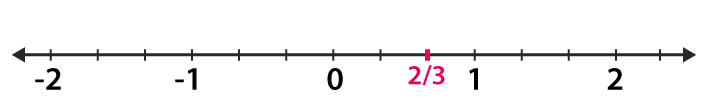(ii) We know that (3/4) is greater than 0 and less than 1.
∴ it lies between 0 and 1. It can be represented on number line as,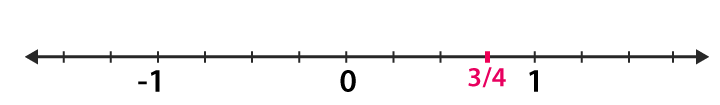(iii) We know that (3/8) is greater than 0 and less than 1.
∴ it lies between 0 and 1. It can be represented on number line as,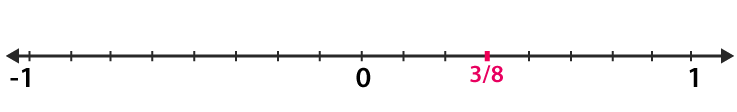(iv) We know that (-5/8) is greater than -1 and less than 0.
∴ it lies between 0 and -1. It can be represented on number line as,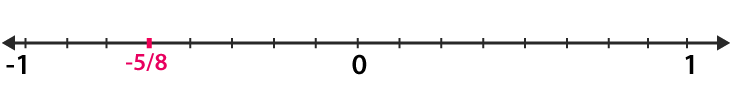(v) We know that (-3/16) is greater than -1 and less than 0.
∴ it lies between 0 and -1. It can be represented on number line as,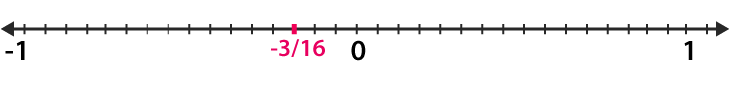(vi) We know that (-7/3) is greater than -3 and less than -2.
∴ it lies between -3 and -2. It can be represented on number line as,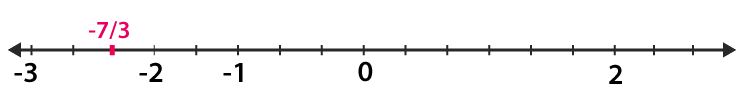(vii) We know that (22/-7) is greater than -4 and less than -3.
∴ it lies between -3 and -4. It can be represented on number line as,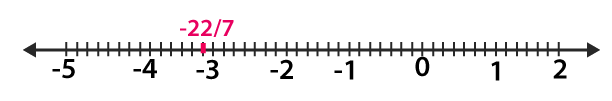(Viii) We know that (-31/3) is greater than -11 and less than -10.
∴ it lies between -10 and -11. It can be represented on number line as,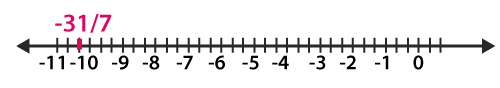2. Which of the two rational numbers in each of the following pairs of rational number is greater?
(i) (-3/8), 0
(ii) (5/2), 0
(iii) (– 4/11), (3/11)
(iv) (– 7/12), (5/- 8)
(v)  (4/-9), (– 3/- 7)
(vi) (– 5/8), (3/- 4)
(vii) (5/9), (-3/- 8)
(viii)  (5/- 8), (-7/12)
Solution:
(i) Given (-3/8), 0
We know that every positive rational number is greater than zero and every negative
rational number is smaller than zero. Thus, – (3/8) > 0
(ii)  Given (5/2), 0
We know that every positive rational number is greater than zero and every negative rational number is smaller than zero. Thus, (5/2) > 0
(iii) Given (– 4/11), (3/11)
We know that every positive rational number is greater than zero and every negative rational number is smaller than zero, also the denominator is same in given question now we have to compare the numerator, thus – 4/11 < 3/11.
(iv) Given (– 7/12), (5/- 8)
Consider (– 7/12)
Multiply both numerator and denominator by 2 then we get
(-7/12) × (2/2) = (-14/24)…… (1)
Now consider (5/-8)
Multiply both numerator and denominator by 3 we get
(5/-8) × (3/3) = (15/-24)…… (2)
The denominator is same in equation (1) and (2) now we have to compare the numerator, thus (– 7/12) > (5/- 8)
(v) Given (4/-9), (– 3/- 7)
Consider (4/-9)
Multiply both numerator and denominator by 7 then we get
(4/-9) × (7/7) = (28/-63)…… (1)
Now consider (-3/-7)
Multiply both numerator and denominator by 9 we get
(-3/-7) × (9/9) = (-27/-63)…… (2)
The denominator is same in equation (1) and (2) now we have to compare the numerator, thus (4/-9) < (– 3/- 7)
(vi) Given (– 5/8), (3/- 4)
Now consider (3/-4)
Multiply both numerator and denominator by 2 we get
(3/-4) × (2/2) = (6/-8)
The denominator is same in above equation now we have to compare the numerator, thus (– 5/8) > (3/- 4)
(vii) Given (5/9), (-3/- 8)
Consider (5/9)
Multiply both numerator and denominator by 8 then we get
(5/9) × (8/8) = (40/72)…… (1)
Now consider (5/-8)
Multiply both numerator and denominator by 9 we get
(-3/-8) × (9/9) = (-27/-72)…… (2)
The denominator is same in equation (1) and (2) now we have to compare the numerator, thus (5/9) > (-3/- 8)
(viii) Given (5/- 8), (-7/12)
Consider (5/-8)
Multiply both numerator and denominator by 3 then we get
(5/-8) × (3/3) = (15/-24)…… (1)
Now consider (-7/12)
Multiply both numerator and denominator by 2 we get
(-7/12) × (2/2) = (-14/24)…… (2)
The denominator is same in equation (1) and (2) now we have to compare the numerator, thus (5/- 8) < (-7/12)
3. Which of the two rational numbers in each of the following pairs of rational numbers is smaller?
(i) (-6/-13), (7/13)
(ii) (16/-5), 3
(iii) (-4/3), (8/-7)
(iv) (-12/5), (-3)
Solution:
(i) Given (-6/-13), (7/13)
Here denominator is same therefore compare the numerator,
Thus (-6/-13) < (7/13)
(ii) Given (16/-5), 3
We know that 3 is a whole number with positive sign
Therefore (16/-5) < 3
(iii) Given (-4/3), (8/-7)
Consider (-4/3)
Multiply both numerator and denominator by 7 then we get
(-4/3) × (7/7) = (-28/21)…… (1)
Now consider (8/-7)
Multiply both numerator and denominator by 3 we get
(8/-7) × (3/3) = (-24/21)…… (2)
The denominator is same in equation (1) and (2) now we have to compare the numerator, thus (-4/3) < (8/-7)
(iv) Given (-12/5), (-3)
Now consider (-3/1)
Multiply both numerator and denominator by 5 we get
(-3/1) × (5/5) = (-15/5)
The denominator is same in above equation, now we have to compare the numerator, thus (-12/5) > (-3)
4. Fill in the blanks by the correct symbol out of >, =, or <: strong="">
(i) (-6/7) …. (7/13)
(ii) (-3/5) …. (-5/6)
(iii) (-2/3) …. (5/-8)
(iv) 0 …. (-2/5)
Solution:
(i) (- 6/7) < (7/13)
Explanation:
Because every positive number is greater than a negative number.
(ii) (-3/5) > (-5/6)
Explanation:
Consider (-3/5)
Multiply both numerator and denominator by 6 then we get
(-3/5) × (6/6) = (-18/30)…… (1)
Now consider (-5/6)
Multiply both numerator and denominator by 5 we get
(-5/6) × (5/5) = (-25/30)…… (2)
The denominator is same in equation (1) and (2) now we have to compare the numerator, thus (-3/5) > (-5/6)
(iii) (-2/3) < (5/-8)
Explanation:
Consider (-2/3)
Multiply both numerator and denominator by 8 then we get
(-2/3) × (8/8) = (-16/24)…… (1)
Now consider (5/-8)
Multiply both numerator and denominator by 3 we get
(5/-8) × (3/3) = (15/-24)…… (2)
The denominator is same in equation (1) and (2) now we have to compare the numerator, thus (-2/3) < (5/-8)
(iv) 0 > (-2/5)
Explanation:
Because every positive number is greater than a negative number
5. Arrange the following rational numbers in ascending order:
(i) (3/5), (-17/-30), (8/-15), (-7/10)
(ii) (-4/9), (5/-12), (7/-18), (2/-3)
Solution:
(i) Given (3/5), (-17/-30), (8/-15), (-7/10)
The LCM of 5, 30, 15 and 10 is 30
Multiplying the numerators and denominators to get the denominator equal to the LCM i.e. 30
Consider (3/5)
Multiply both numerator and denominator by 6, then we get
(3/5) × (6/6) = (18/30) ….. (1)
Consider (8/-15)
Multiply both numerator and denominator by 2, then we get
(8/-15) × (2/2) = (16/-30) ….. (2)
Consider (-7/10)
Multiply both numerator and denominator by 3, then we get
(-7/10) × (3/3) = (-21/30) ….. (3)
In the above equation, denominators are same
Now on comparing the ascending order is:
(-7/10) < (8/-15) < (-17/30) < (3/5)
(ii) Given (-4/9), (5/-12), (7/-18), (2/-3)
The LCM of 9, 12, 18 and 3 is 36
Multiplying the numerators and denominators to get the denominator equal to the LCM i.e. 36
Consider (-4/9)
Multiply both numerator and denominator by 4, then we get
(-4/9) × (4/4) = (-16/36) ….. (1)
Consider (5/-12)
Multiply both numerator and denominator by 3, then we get
(5/-12) × (3/3) = (15/-36) ….. (2)
Consider (7/-18)
Multiply both numerator and denominator by 2, then we get
(7/-18) × (2/2) = (14/-36) ….. (3)
Consider (2/-3)
Multiply both numerator and denominator by 12, then we get
(2/-3) × (12/12) = (24/-36) ….. (4)
In the above equation, denominators are same
Now on comparing the ascending order is:
(2/-3) < ((-4/9) < (5/-12) < (7/-18)
6. Arrange the following rational numbers in descending order:
(i) (7/8), (64/16), (39/-12), (5/-4), (140/28)
(ii) (-3/10), (17/-30), (7/-15), (-11/20)
Solution:
(i) Given (7/8), (64/16), (39/-12), (5/-4), (140/28)
The LCM of 8, 16, 12, 4 and 28 is 336
Multiplying the numerators and denominators to get the denominator equal to the LCM i.e. 336
Consider (7/8)
Multiply both numerator and denominator by 42, then we get
(7/8) × (42/42) = (294/336) ….. (1)
Consider (64/16)
Multiply both numerator and denominator by 21, then we get
(64/16) × (21/21) = (1344/336) ….. (2)
Consider (39/-12)
Multiply both numerator and denominator by 28, then we get
(39/-12) × (28/28) = (-1008/336) ….. (3)
Consider (5/-4)
Multiply both numerator and denominator by 84, then we get
(5/-4) × (84/84) = (-420/336) ….. (4)
In the above equation, denominators are same
Now on comparing the descending order is:
(140/28) > (64/16) > (7/8) > (5/-4) > (36/-12)
(ii) Given (-3/10), (17/-30), (7/-15), (-11/20)
The LCM of 10, 30, 15 and 20 is 60
Multiplying the numerators and denominators to get the denominator equal to the LCM i.e. 60
Consider (-3/10)
Multiply both numerator and denominator by 6, then we get
(-3/10) × (6/6) = (-18/60) ….. (1)
Consider (17/-30)
Multiply both numerator and denominator by 2, then we get
(17/-30) × (2/2) = (34/-60) ….. (2)
Consider (7/-15)
Multiply both numerator and denominator by 4, then we get
(7/-15) × (4/4) = (28/-60) ….. (3)
In the above equation, denominators are same
Now on comparing the descending order is:
(-3/10) > (7/-15) > (-11/20) > (17/20)
7. Which of the following statements are true:
(i) The rational number (29/23) lies to the left of zero on the number line.
(ii) The rational number (-12/-17) lies to the left of zero on the number line.
(iii) The rational number (3/4) lies to the right of zero on the number line.
(iv) The rational number (-12/-5) and (- 7/17) are on the opposite side of zero on the number line.
(v) The rational number (-2/15) and (7/-31) are on the opposite side of zero on the number line.
(vi) The rational number (- 3/-5) is on the right of (- 4/7) on the number line.
Solution:
(i) False
Explanation:
It lies to the right of zero because it is a positive number.
(ii) False
Explanation:
It lies to the right of zero because it is a positive number.
(iii) True
Explanation:
Always positive number lie on the right of zero
(iv) True
Explanation:
Because they are of opposite sign
(v) False
Explanation:
Because they both are of same sign
(vi) True
Explanation:
They both are of opposite signs and positive number is greater than the negative number. Thus, it is on the right of the negative number.
Courtesy : CBSE